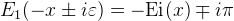Computes the principal value of the exponential integral.

Namespace:  Meta.Numerics.Functions
Assembly:  Meta.Numerics (in Meta.Numerics.dll) Version: 4.1.4Syntax
```public static double IntegralEi(
double x
)```

#### Parameters

x
Type: SystemDouble
The argument, which must be non-negative.

#### Return Value

Type: Double
The value of Ei(x).Exceptions
ExceptionCondition
ArgumentOutOfRangeExceptionx is negative.Remarks

The function Ei(x) appears in the evaluation of some indefinite integrals involving exponents and in number theory in the approximation li(x) = Ei(ln x) to the cumulative distribution of primes.

Ei(x) is related to the real part of E1(x) for negative arguments by:To compute Ei(z) in the entire complex plane, use Ein(Complex).See Also